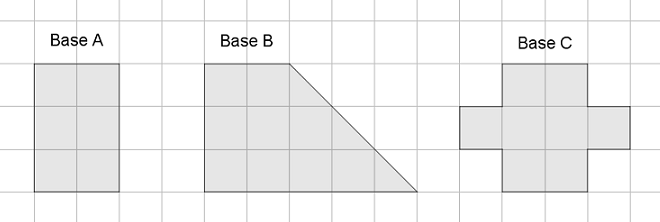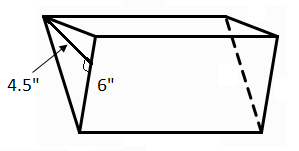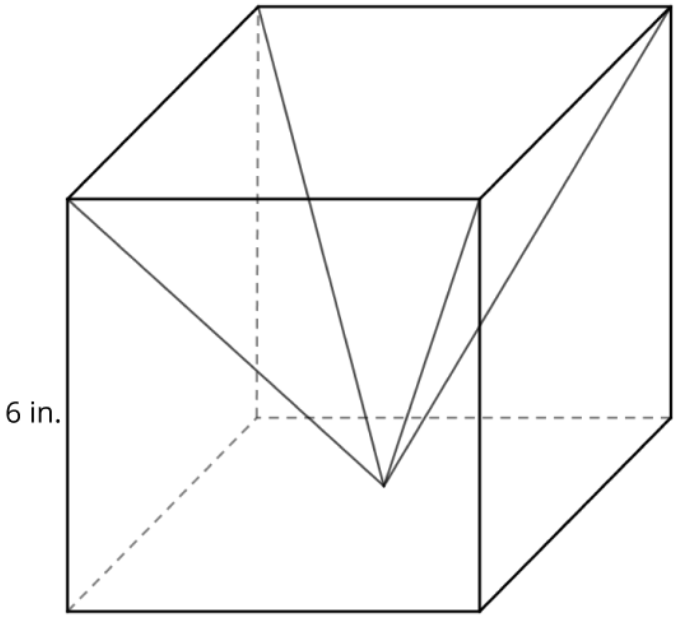Match Fishtank is now Fishtank Learning!

# Geometry

## Objective

Find the volume of right prisms and pyramids.

## Common Core Standards

### Core Standards

?

• 7.G.B.6 — Solve real-world and mathematical problems involving area, volume and surface area of two- and three-dimensional objects composed of triangles, quadrilaterals, polygons, cubes, and right prisms.

?

• 6.G.A.2

• 5.MD.C.5

## Criteria for Success

?

1. Understand that the area of the base of a prism multiplied by the height of the prism gives the volume of the prism.
2. Use the formula for volume to find missing measurements of a prism.
3. Understand that the volume of a pyramid is ${1\over 3}$ the volume of a prism with the same base.

## Tips for Teachers

?

Lessons 19 and 20 engage students in finding the volume of prisms and pyramids. Students use their equation skills to manipulate the volume formula to find missing measurements. Lesson 19 focuses on mathematical problems and Lesson 20 focuses on solving real-world problems. In Lesson 21, students will compare volume to surface area in real-world contexts.

#### Remote Learning Guidance

If you need to adapt or shorten this lesson for remote learning, we suggest prioritizing Anchor Problems 2 and 3 (benefit from worked examples). As AP3 is a discovery problem, if live discussion or reflection of the problem were possible, it would allow for students to arrive at the conclusion on their own. Find more guidance on adapting our math curriculum for remote learning here.

#### Fishtank Plus

• Problem Set
• Student Handout Editor
• Vocabulary Package

## Anchor Problems

?

### Problem 1

The three shapes below represent the bases of three different prisms. Each grid is 1 unit x 1 unit, and each prism has a height of 10 units.What is the volume of each prism?

### Problem 2

A triangular prism has the measurements shown below.If the volume of the prism is 270 cubic inches, what is the height of the prism?

### Problem 3

Investigate the relationship between the volume of a prism and a pyramid with the same base.

1. Watch the YouTube video Volume of Three Square Pyramids Fitting into a Cube.
2. What is the relationship between the volume of the prism and the volume of the pyramid?
3. What formula can you use to find the volume of a pyramid?
4. Find the volume of a rectangular pyramid with height 5 cm and base measuring 8 cm × 6 cm.

## Problem Set

?The following resources include problems and activities aligned to the objective of the lesson that can be used to create your own problem set.